# Powers of Two Trace Ex Print powers of

• Slides: 33Powers of Two: Trace Ex. Print powers of 2 that are 2 N. Increment i from 0 to N. Double v each time. n n int i = 0; int v = 1; while (i <= N) { System. out. println(i + " " + v); i = i + 1; v = 2 * v; } N = 6 1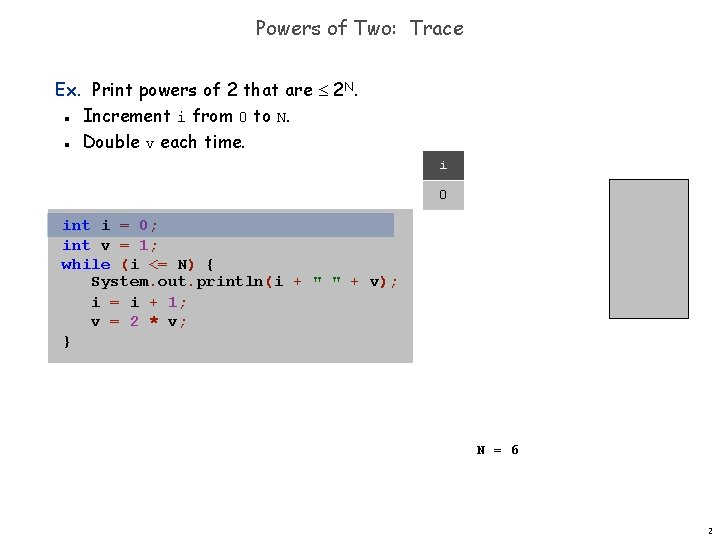Powers of Two: Trace Ex. Print powers of 2 that are 2 N. Increment i from 0 to N. Double v each time. n n i 0 int i = 0; int v = 1; while (i <= N) { System. out. println(i + " " + v); i = i + 1; v = 2 * v; } N = 6 2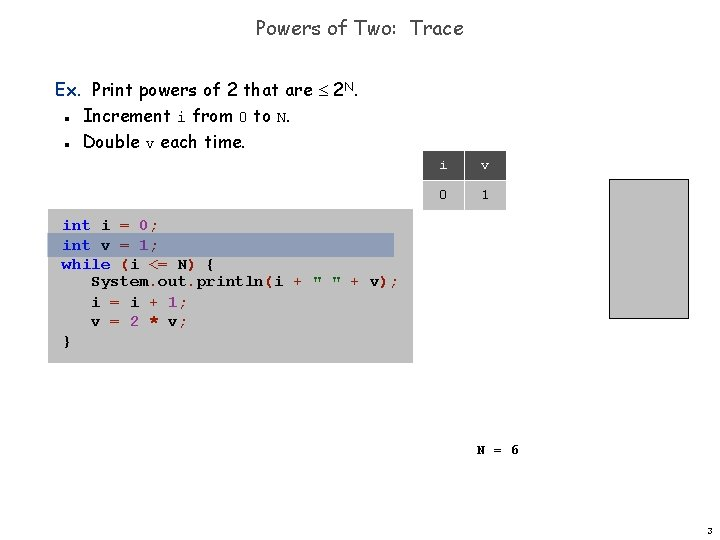Powers of Two: Trace Ex. Print powers of 2 that are 2 N. Increment i from 0 to N. Double v each time. n n i v 0 1 int i = 0; int v = 1; while (i <= N) { System. out. println(i + " " + v); i = i + 1; v = 2 * v; } N = 6 3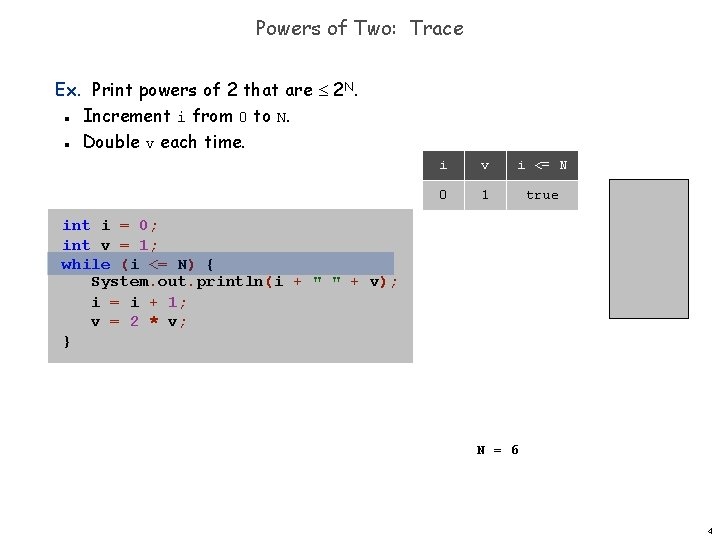Powers of Two: Trace Ex. Print powers of 2 that are 2 N. Increment i from 0 to N. Double v each time. n n i v i <= N 0 1 true int i = 0; int v = 1; while (i <= N) { System. out. println(i + " " + v); i = i + 1; v = 2 * v; } N = 6 4Powers of Two: Trace Ex. Print powers of 2 that are 2 N. Increment i from 0 to N. Double v each time. n n i v i <= N 0 1 true 0 1 int i = 0; int v = 1; while (i <= N) { System. out. println(i + " " + v); i = i + 1; v = 2 * v; } N = 6 5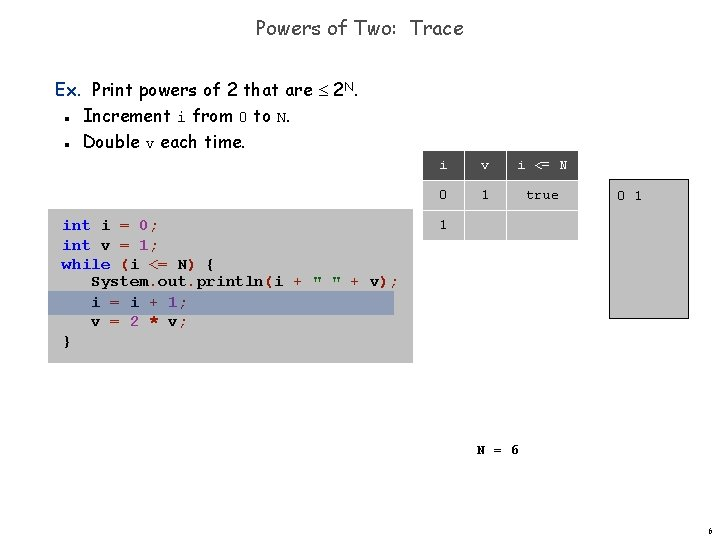Powers of Two: Trace Ex. Print powers of 2 that are 2 N. Increment i from 0 to N. Double v each time. n n int i = 0; int v = 1; while (i <= N) { System. out. println(i + " " + v); i = i + 1; v = 2 * v; } i v i <= N 0 1 true 0 1 1 N = 6 6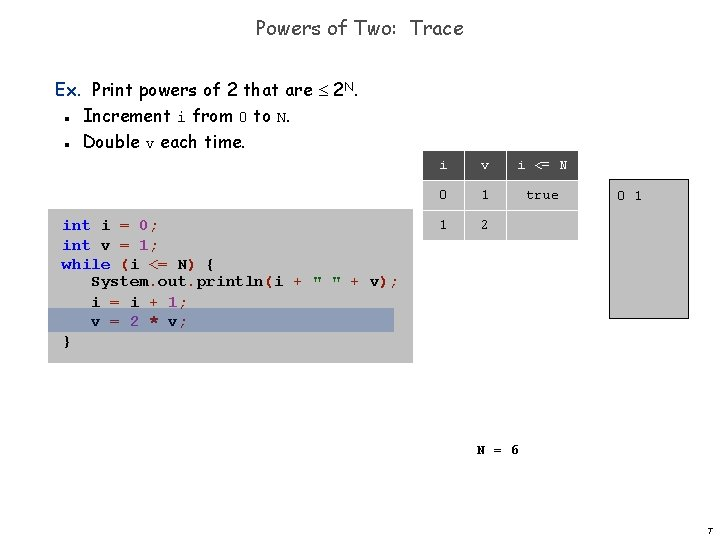Powers of Two: Trace Ex. Print powers of 2 that are 2 N. Increment i from 0 to N. Double v each time. n n int i = 0; int v = 1; while (i <= N) { System. out. println(i + " " + v); i = i + 1; v = 2 * v; } i v i <= N 0 1 true 1 2 0 1 N = 6 7Powers of Two: Trace Ex. Print powers of 2 that are 2 N. Increment i from 0 to N. Double v each time. n n int i = 0; int v = 1; while (i <= N) { System. out. println(i + " " + v); i = i + 1; v = 2 * v; } i v i <= N 0 1 true 1 2 true 0 1 N = 6 8Powers of Two: Trace Ex. Print powers of 2 that are 2 N. Increment i from 0 to N. Double v each time. n n int i = 0; int v = 1; while (i <= N) { System. out. println(i + " " + v); i = i + 1; v = 2 * v; } i v i <= N 0 1 true 1 2 true 0 1 1 2 N = 6 9Powers of Two: Trace Ex. Print powers of 2 that are 2 N. Increment i from 0 to N. Double v each time. n n int i = 0; int v = 1; while (i <= N) { System. out. println(i + " " + v); i = i + 1; v = 2 * v; } i v i <= N 0 1 true 1 2 true 0 1 1 2 2 N = 6 10Powers of Two: Trace Ex. Print powers of 2 that are 2 N. Increment i from 0 to N. Double v each time. n n int i = 0; int v = 1; while (i <= N) { System. out. println(i + " " + v); i = i + 1; v = 2 * v; } i v i <= N 0 1 true 1 2 true 2 4 0 1 1 2 N = 6 11Powers of Two: Trace Ex. Print powers of 2 that are 2 N. Increment i from 0 to N. Double v each time. n n int i = 0; int v = 1; while (i <= N) { System. out. println(i + " " + v); i = i + 1; v = 2 * v; } i v i <= N 0 1 true 1 2 true 2 4 true 0 1 1 2 N = 6 12Powers of Two: Trace Ex. Print powers of 2 that are 2 N. Increment i from 0 to N. Double v each time. n n int i = 0; int v = 1; while (i <= N) { System. out. println(i + " " + v); i = i + 1; v = 2 * v; } i v i <= N 0 1 true 1 2 true 2 4 true 0 1 1 2 2 4 N = 6 13Powers of Two: Trace Ex. Print powers of 2 that are 2 N. Increment i from 0 to N. Double v each time. n n int i = 0; int v = 1; while (i <= N) { System. out. println(i + " " + v); i = i + 1; v = 2 * v; } i v i <= N 0 1 true 1 2 true 2 4 true 0 1 1 2 2 4 3 N = 6 14Powers of Two: Trace Ex. Print powers of 2 that are 2 N. Increment i from 0 to N. Double v each time. n n int i = 0; int v = 1; while (i <= N) { System. out. println(i + " " + v); i = i + 1; v = 2 * v; } i v i <= N 0 1 true 1 2 true 2 4 true 3 8 0 1 1 2 2 4 N = 6 15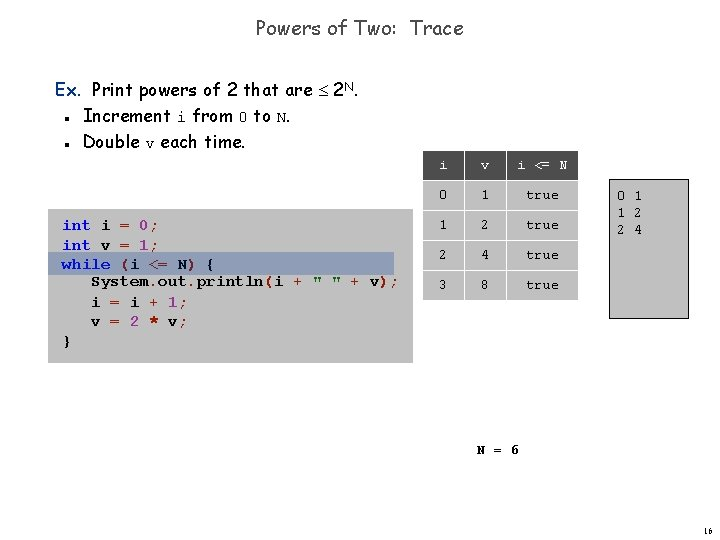Powers of Two: Trace Ex. Print powers of 2 that are 2 N. Increment i from 0 to N. Double v each time. n n int i = 0; int v = 1; while (i <= N) { System. out. println(i + " " + v); i = i + 1; v = 2 * v; } i v i <= N 0 1 true 1 2 true 2 4 true 3 8 true 0 1 1 2 2 4 N = 6 16Powers of Two: Trace Ex. Print powers of 2 that are 2 N. Increment i from 0 to N. Double v each time. n n int i = 0; int v = 1; while (i <= N) { System. out. println(i + " " + v); i = i + 1; v = 2 * v; } i v i <= N 0 1 true 1 2 true 2 4 true 3 8 true 0 1 2 3 1 2 4 8 N = 6 17Powers of Two: Trace Ex. Print powers of 2 that are 2 N. Increment i from 0 to N. Double v each time. n n int i = 0; int v = 1; while (i <= N) { System. out. println(i + " " + v); i = i + 1; v = 2 * v; } i v i <= N 0 1 true 1 2 true 2 4 true 3 8 true 0 1 2 3 1 2 4 8 4 N = 6 18Powers of Two: Trace Ex. Print powers of 2 that are 2 N. Increment i from 0 to N. Double v each time. n n int i = 0; int v = 1; while (i <= N) { System. out. println(i + " " + v); i = i + 1; v = 2 * v; } i v i <= N 0 1 true 1 2 true 2 4 true 3 8 true 4 16 0 1 2 3 1 2 4 8 N = 6 19Powers of Two: Trace Ex. Print powers of 2 that are 2 N. Increment i from 0 to N. Double v each time. n n int i = 0; int v = 1; while (i <= N) { System. out. println(i + " " + v); i = i + 1; v = 2 * v; } i v i <= N 0 1 true 1 2 true 2 4 true 3 8 true 4 16 true 0 1 2 3 1 2 4 8 N = 6 20Powers of Two: Trace Ex. Print powers of 2 that are 2 N. Increment i from 0 to N. Double v each time. n n int i = 0; int v = 1; while (i <= N) { System. out. println(i + " " + v); i = i + 1; v = 2 * v; } i v i <= N 0 1 true 1 2 true 2 4 true 3 8 true 4 16 true 0 1 2 3 4 1 2 4 8 16 N = 6 21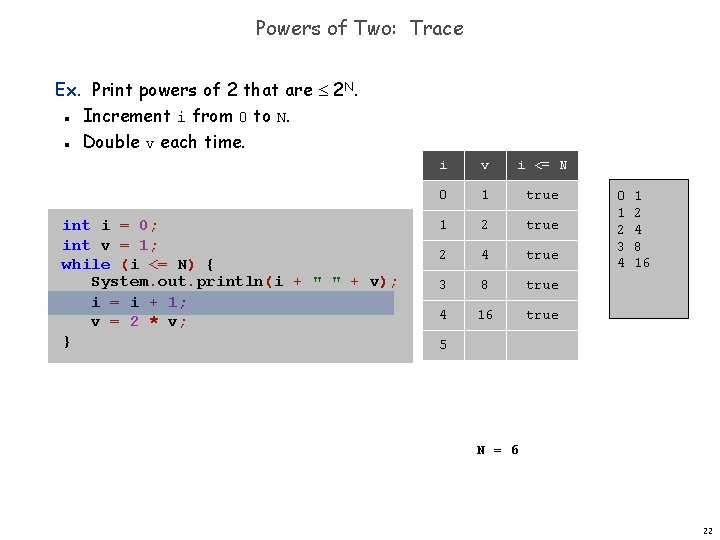Powers of Two: Trace Ex. Print powers of 2 that are 2 N. Increment i from 0 to N. Double v each time. n n int i = 0; int v = 1; while (i <= N) { System. out. println(i + " " + v); i = i + 1; v = 2 * v; } i v i <= N 0 1 true 1 2 true 2 4 true 3 8 true 4 16 true 0 1 2 3 4 1 2 4 8 16 5 N = 6 22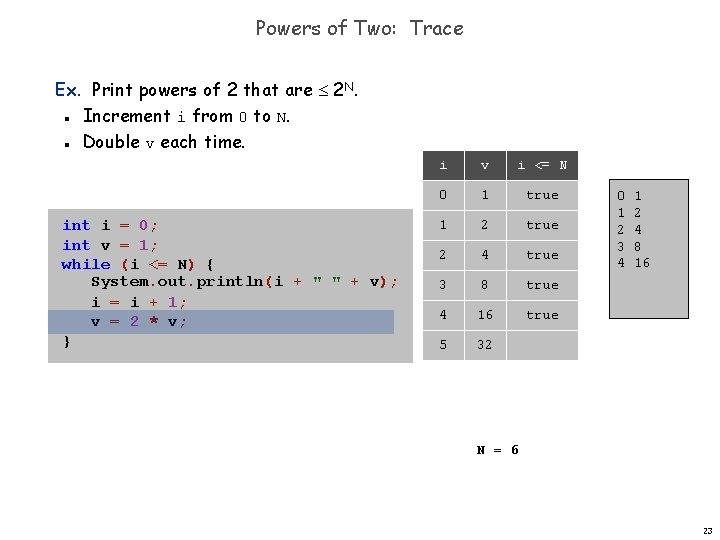Powers of Two: Trace Ex. Print powers of 2 that are 2 N. Increment i from 0 to N. Double v each time. n n int i = 0; int v = 1; while (i <= N) { System. out. println(i + " " + v); i = i + 1; v = 2 * v; } i v i <= N 0 1 true 1 2 true 2 4 true 3 8 true 4 16 true 5 32 0 1 2 3 4 1 2 4 8 16 N = 6 23Powers of Two: Trace Ex. Print powers of 2 that are 2 N. Increment i from 0 to N. Double v each time. n n int i = 0; int v = 1; while (i <= N) { System. out. println(i + " " + v); i = i + 1; v = 2 * v; } i v i <= N 0 1 true 1 2 true 2 4 true 3 8 true 4 16 true 5 32 true 0 1 2 3 4 1 2 4 8 16 N = 6 24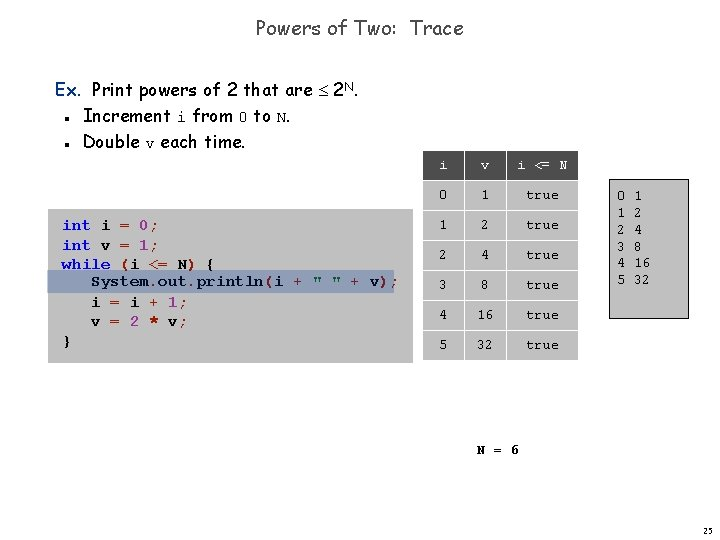Powers of Two: Trace Ex. Print powers of 2 that are 2 N. Increment i from 0 to N. Double v each time. n n int i = 0; int v = 1; while (i <= N) { System. out. println(i + " " + v); i = i + 1; v = 2 * v; } i v i <= N 0 1 true 1 2 true 2 4 true 3 8 true 4 16 true 5 32 true 0 1 2 3 4 5 1 2 4 8 16 32 N = 6 25Powers of Two: Trace Ex. Print powers of 2 that are 2 N. Increment i from 0 to N. Double v each time. n n int i = 0; int v = 1; while (i <= N) { System. out. println(i + " " + v); i = i + 1; v = 2 * v; } i v i <= N 0 1 true 1 2 true 2 4 true 3 8 true 4 16 true 5 32 true 0 1 2 3 4 5 1 2 4 8 16 32 6 N = 6 26Powers of Two: Trace Ex. Print powers of 2 that are 2 N. Increment i from 0 to N. Double v each time. n n int i = 0; int v = 1; while (i <= N) { System. out. println(i + " " + v); i = i + 1; v = 2 * v; } i v i <= N 0 1 true 1 2 true 2 4 true 3 8 true 4 16 true 5 32 true 6 64 0 1 2 3 4 5 1 2 4 8 16 32 N = 6 27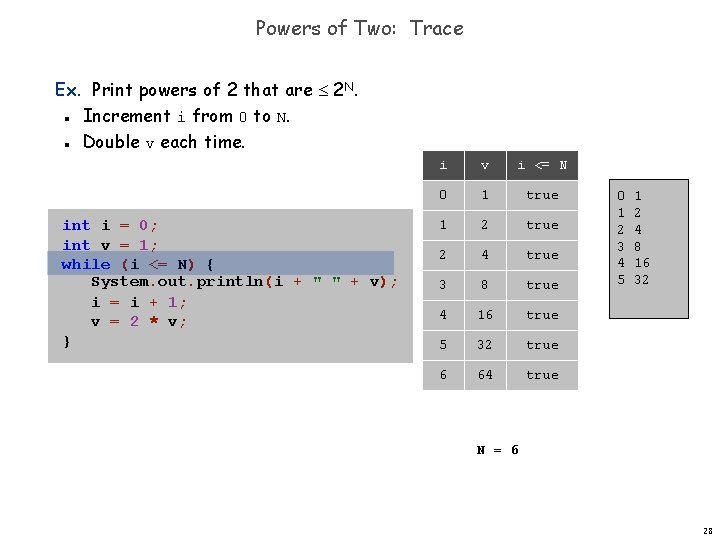Powers of Two: Trace Ex. Print powers of 2 that are 2 N. Increment i from 0 to N. Double v each time. n n int i = 0; int v = 1; while (i <= N) { System. out. println(i + " " + v); i = i + 1; v = 2 * v; } i v i <= N 0 1 true 1 2 true 2 4 true 3 8 true 4 16 true 5 32 true 6 64 true 0 1 2 3 4 5 1 2 4 8 16 32 N = 6 28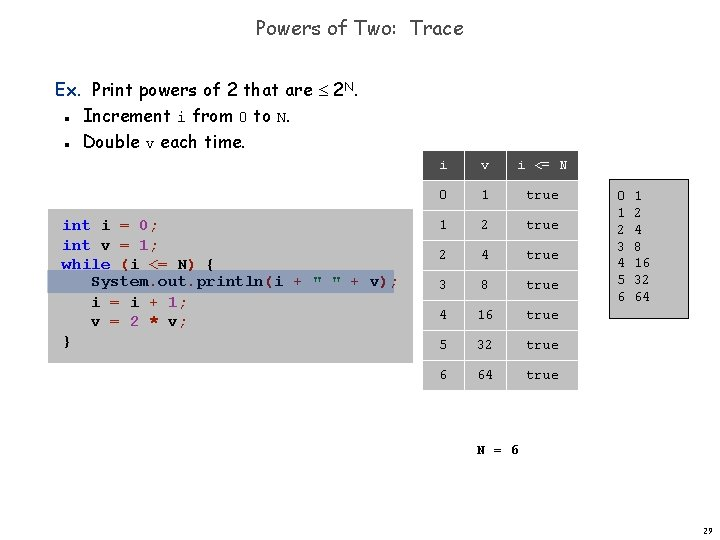Powers of Two: Trace Ex. Print powers of 2 that are 2 N. Increment i from 0 to N. Double v each time. n n int i = 0; int v = 1; while (i <= N) { System. out. println(i + " " + v); i = i + 1; v = 2 * v; } i v i <= N 0 1 true 1 2 true 2 4 true 3 8 true 4 16 true 5 32 true 6 64 true 0 1 2 3 4 5 6 1 2 4 8 16 32 64 N = 6 29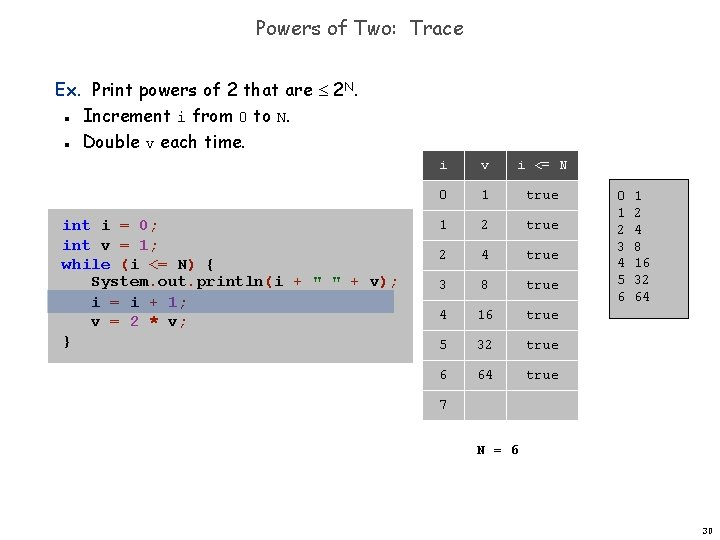Powers of Two: Trace Ex. Print powers of 2 that are 2 N. Increment i from 0 to N. Double v each time. n n int i = 0; int v = 1; while (i <= N) { System. out. println(i + " " + v); i = i + 1; v = 2 * v; } i v i <= N 0 1 true 1 2 true 2 4 true 3 8 true 4 16 true 5 32 true 6 64 true 0 1 2 3 4 5 6 1 2 4 8 16 32 64 7 N = 6 30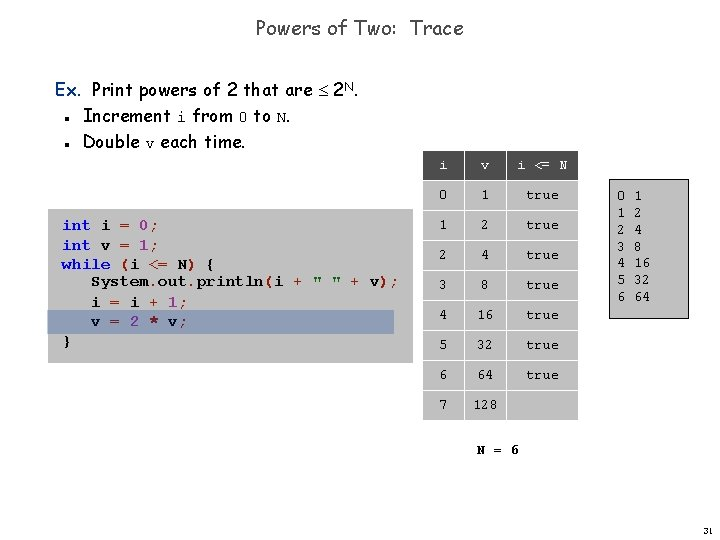Powers of Two: Trace Ex. Print powers of 2 that are 2 N. Increment i from 0 to N. Double v each time. n n int i = 0; int v = 1; while (i <= N) { System. out. println(i + " " + v); i = i + 1; v = 2 * v; } i v i <= N 0 1 true 1 2 true 2 4 true 3 8 true 4 16 true 5 32 true 6 64 true 7 128 0 1 2 3 4 5 6 1 2 4 8 16 32 64 N = 6 31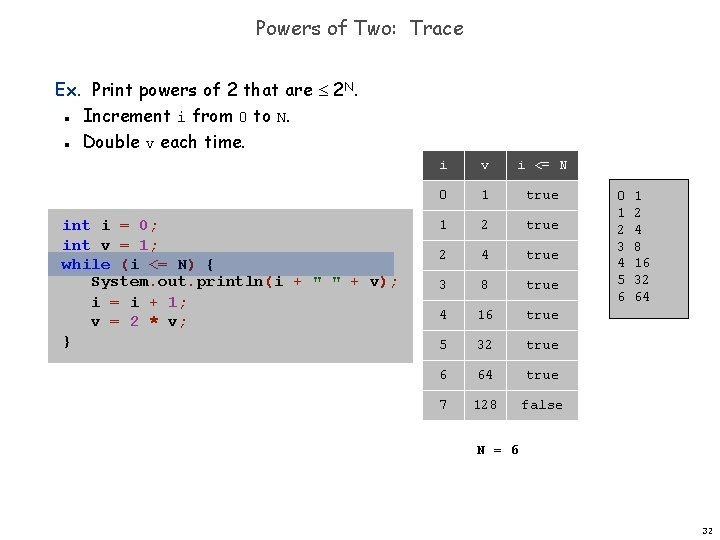Powers of Two: Trace Ex. Print powers of 2 that are 2 N. Increment i from 0 to N. Double v each time. n n int i = 0; int v = 1; while (i <= N) { System. out. println(i + " " + v); i = i + 1; v = 2 * v; } i v i <= N 0 1 true 1 2 true 2 4 true 3 8 true 4 16 true 5 32 true 6 64 true 7 128 false 0 1 2 3 4 5 6 1 2 4 8 16 32 64 N = 6 32Powers of Two: Trace Ex. Print powers of 2 that are 2 N. Increment i from 0 to N. Double v each time. n n int i = 0; int v = 1; while (i <= N) { System. out. println(i + " " + v); i = i + 1; v = 2 * v; } i v i <= N 0 1 true 1 2 true 2 4 true 3 8 true 4 16 true 5 32 true 6 64 true 7 128 false 0 1 2 3 4 5 6 1 2 4 8 16 32 64 N = 6 33